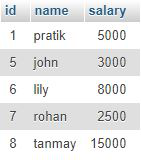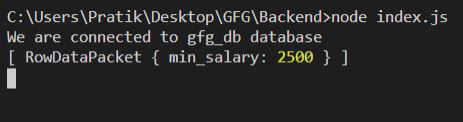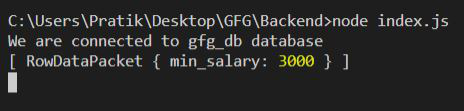Related Articles
NodeJS MySQL Min() Function
• Last Updated : 18 Feb, 2021

We will be using Min() function in MySQL to get Minimum value from some particular column.

Syntax:

`MIN(column_name)`

Parameters: MIN() function accepts a single parameter as mentioned above and described below.

• column_name: Column’s name from which we have to return the min value.

Return Value: MIN() function returns the minimum value from the particular column in the table.

Module Installation: Install the MySQL module using the following command.

`npm install mysql`

Database: Our SQL publishers table preview with sample data is shown below.Example 1:

## index.js

 `const mysql = require(``"mysql"``);`` ` `let db_con  = mysql.createConnection({``    ``host: ``"localhost"``,``    ``user: ``"root"``,``    ``password: ``''``,``    ``database: ``'gfg_db'``});`` ` `db_con.connect((err) => {``    ``if` `(err) {``      ``console.log(``"Database Connection Failed !!!"``, err);``      ``return``;``    ``}`` ` `    ``console.log(``"We are connected to gfg_db database"``);`` ` `    ``// Here is the query``    ``let query = ``"SELECT MIN(salary) AS min_salary FROM publishers"``;`` ` `    ``db_con.query(query, (err, rows) => {``        ``if``(err) ``throw` `err;`` ` `        ``console.log(rows);``    ``});``});`

Output:Example 2:

## index.js

 `const mysql = require(``"mysql"``);`` ` `let db_con  = mysql.createConnection({``    ``host: ``"localhost"``,``    ``user: ``"root"``,``    ``password: ``''``,``    ``database: ``'gfg_db'``});`` ` `db_con.connect((err) => {``    ``if` `(err) {``      ``console.log(``"Database Connection Failed !!!"``, err);``      ``return``;``    ``}`` ` `    ``console.log(``"We are connected to gfg_db database"``);`` ` `    ``// Here is the query``    ``let query = ``"SELECT MIN(salary) AS min_salary ``                 ``FROM publishers WHERE id < 7"``;`` ` `    ``db_con.query(query, (err, rows) => {``        ``if``(err) ``throw` `err;`` ` `        ``console.log(rows);``    ``});``});`

Output:Attention reader! Don’t stop learning now. Get hold of all the important DSA concepts with the DSA Self Paced Course at a student-friendly price and become industry ready.

My Personal Notes arrow_drop_up